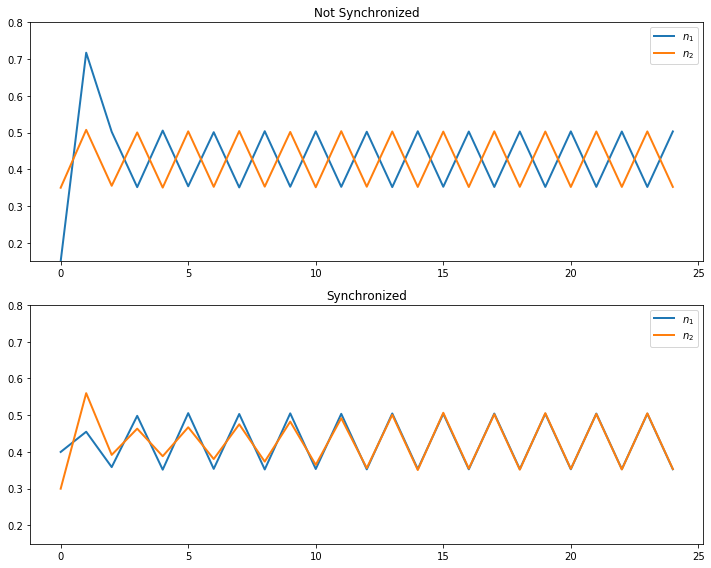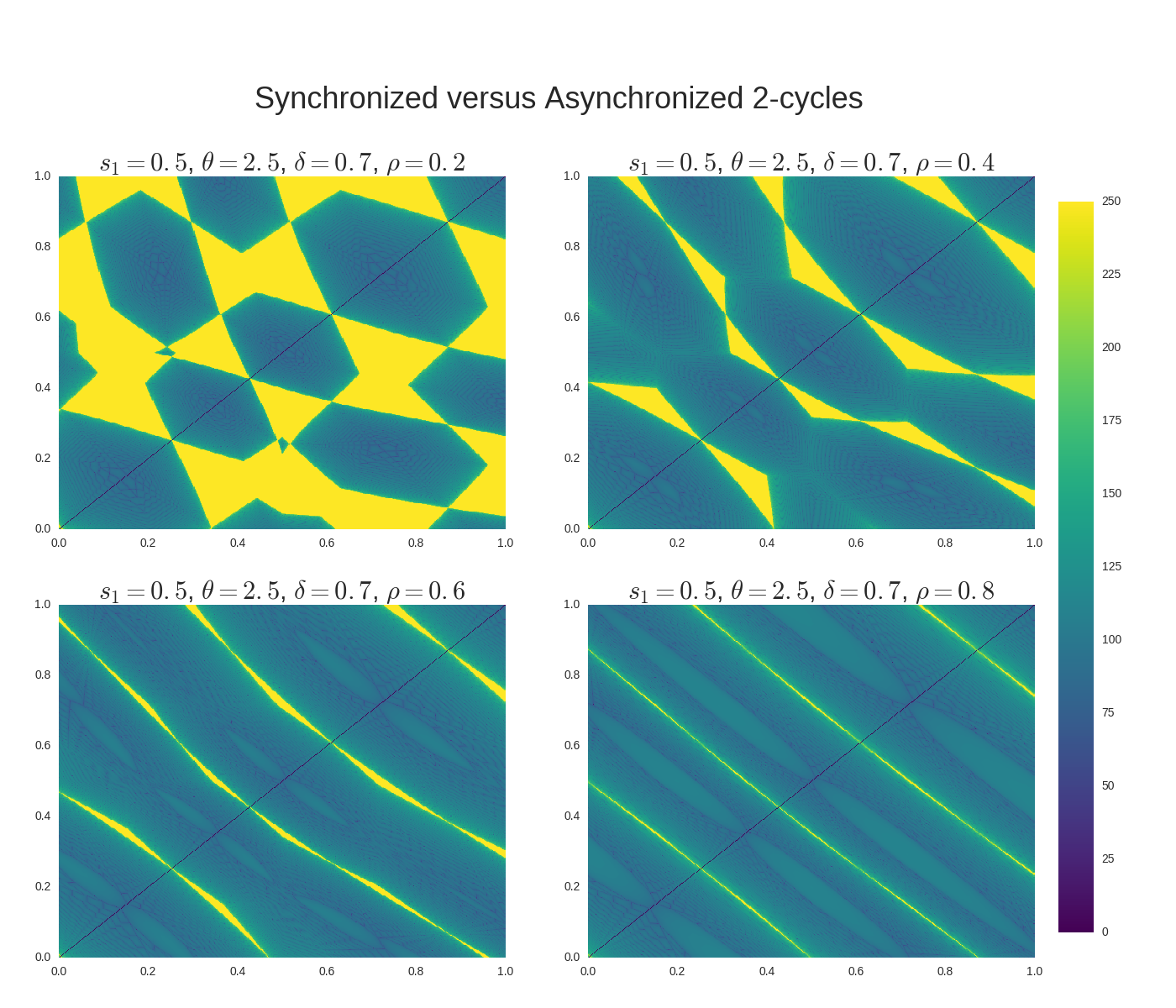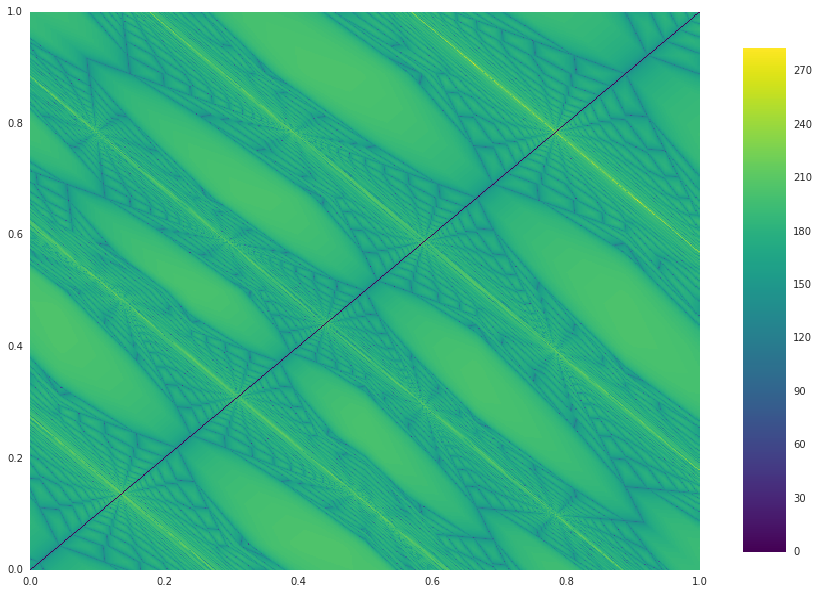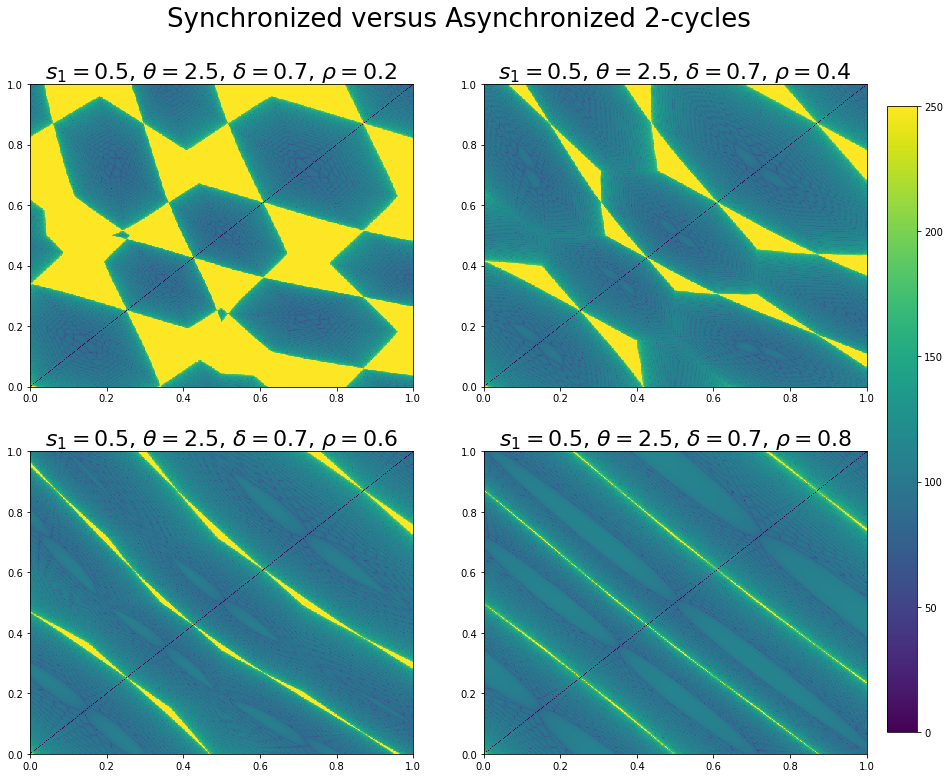# Globalization and Cycles¶

## Overview¶

In this lecture, we review the paper Globalization and Synchronization of Innovation Cycles by Kiminori Matsuyama, Laura Gardini and Iryna Sushko.

This model helps us understand several interesting stylized facts about the world economy.

One of these is synchronized business cycles across different countries.

Most existing models that generate synchronized business cycles do so by assumption, since they tie output in each country to a common shock.

They also fail to explain certain features of the data, such as the fact that the degree of synchronization tends to increase with trade ties.

By contrast, in the model we consider in this lecture, synchronization is both endogenous and increasing with the extent of trade integration.

In particular, as trade costs fall and international competition increases, innovation incentives become aligned and countries synchronize their innovation cycles.

In :
import numpy as np
import matplotlib.pyplot as plt
%matplotlib inline
import seaborn as sns
from numba import jit, vectorize
from ipywidgets import interact


### Background¶

The model builds on work by Judd [Jud85], Deneckner and Judd [DJ92] and Helpman and Krugman [HK85] by developing a two-country model with trade and innovation.

On the technical side, the paper introduces the concept of coupled oscillators to economic modeling.

As we will see, coupled oscillators arise endogenously within the model.

Below we review the model and replicate some of the results on synchronization of innovation across countries.

## Key Ideas¶

It is helpful to begin with an overview of the mechanism.

### Innovation Cycles¶

As discussed above, two countries produce and trade with each other.

In each country, firms innovate, producing new varieties of goods and, in doing so, receiving temporary monopoly power.

Imitators follow and, after one period of monopoly, what had previously been new varieties now enter competitive production.

Firms have incentives to innovate and produce new goods when the mass of varieties of goods currently in production is relatively low.

In addition, there are strategic complementarities in the timing of innovation.

Firms have incentives to innovate in the same period, so as to avoid competing with substitutes that are competitively produced.

This leads to temporal clustering in innovations in each country.

After a burst of innovation, the mass of goods currently in production increases.

However, goods also become obsolete, so that not all survive from period to period.

This mechanism generates a cycle, where the mass of varieties increases through simultaneous innovation and then falls through obsolescence.

### Synchronization¶

In the absence of trade, the timing of innovation cycles in each country is decoupled.

This will be the case when trade costs are prohibitively high.

If trade costs fall, then goods produced in each country penetrate each other’s markets.

As illustrated below, this leads to synchronization of business cycles across the two countries.

## Model¶

Let’s write down the model more formally.

(The treatment is relatively terse since full details can be found in the original paper)

Time is discrete with $t = 0, 1, \dots$.

There are two countries indexed by $j$ or $k$.

In each country, a representative household inelastically supplies $L_j$ units of labor at wage rate $w_{j, t}$.

Without loss of generality, it is assumed that $L_{1} \geq L_{2}$.

Households consume a single nontradeable final good which is produced competitively.

Its production involves combining two types of tradeable intermediate inputs via

$$Y_{k, t} = C_{k, t} = \left( \frac{X^o_{k, t}}{1 - \alpha} \right)^{1-\alpha} \left( \frac{X_{k, t}}{\alpha} \right)^{\alpha}$$

Here $X^o_{k, t}$ is a homogeneous input which can be produced from labor using a linear, one-for-one technology.

It is freely tradeable, competitively supplied, and homogeneous across countries.

By choosing the price of this good as numeraire and assuming both countries find it optimal to always produce the homogeneous good, we can set $w_{1, t} = w_{2, t} = 1$.

The good $X_{k, t}$ is a composite, built from many differentiated goods via

$$X_{k, t}^{1 - \frac{1}{\sigma}} = \int_{\Omega_t} \left[ x_{k, t}(\nu) \right]^{1 - \frac{1}{\sigma}} d \nu$$

Here $x_{k, t}(\nu)$ is the total amount of a differentiated good $\nu \in \Omega_t$ that is produced.

The parameter $\sigma > 1$ is the direct partial elasticity of substitution between a pair of varieties and $\Omega_t$ is the set of varieties available in period $t$.

We can split the varieties into those which are supplied competitively and those supplied monopolistically; that is, $\Omega_t = \Omega_t^c + \Omega_t^m$.

### Prices¶

Demand for differentiated inputs is

$$x_{k, t}(\nu) = \left( \frac{p_{k, t}(\nu)}{P_{k, t}} \right)^{-\sigma} \frac{\alpha L_k}{P_{k, t}}$$

Here

• $p_{k, t}(\nu)$ is the price of the variety $\nu$ and
• $P_{k, t}$ is the price index for differentiated inputs in $k$, defined by
$$\left[ P_{k, t} \right]^{1 - \sigma} = \int_{\Omega_t} [p_{k, t}(\nu) ]^{1-\sigma} d\nu$$

The price of a variety also depends on the origin, $j$, and destination, $k$, of the goods because shipping varieties between countries incurs an iceberg trade cost $\tau_{j,k}$.

Thus the effective price in country $k$ of a variety $\nu$ produced in country $j$ becomes $p_{k, t}(\nu) = \tau_{j,k} \, p_{j, t}(\nu)$.

Using these expressions, we can derive the total demand for each variety, which is

$$D_{j, t}(\nu) = \sum_k \tau_{j, k} x_{k, t}(\nu) = \alpha A_{j, t}(p_{j, t}(\nu))^{-\sigma}$$

where

$$A_{j, t} := \sum_k \frac{\rho_{j, k} L_{k}}{(P_{k, t})^{1 - \sigma}} \quad \text{and} \quad \rho_{j, k} = (\tau_{j, k})^{1 - \sigma} \leq 1$$

It is assumed that $\tau_{1,1} = \tau_{2,2} = 1$ and $\tau_{1,2} = \tau_{2,1} = \tau$ for some $\tau > 1$, so that

$$\rho_{1,2} = \rho_{2,1} = \rho := \tau^{1 - \sigma} < 1$$

The value $\rho \in [0, 1)$ is a proxy for the degree of globalization.

Producing one unit of each differentiated variety requires $\psi$ units of labor, so the marginal cost is equal to $\psi$ for $\nu \in \Omega_{j, t}$.

Additionally, all competitive varieties will have the same price (because of equal marginal cost), which means that, for all $\nu \in \Omega^c$,

$$p_{j, t}(\nu) = p_{j, t}^c := \psi \quad \text{and} \quad D_{j, t} = y_{j, t}^c := \alpha A_{j, t} (p_{j, t}^c)^{-\sigma}$$

Monopolists will have the same marked-up price, so, for all $\nu \in \Omega^m$ ,

$$p_{j, t}(\nu) = p_{j, t}^m := \frac{\psi }{1 - \frac{1}{\sigma}} \quad \text{and} \quad D_{j, t} = y_{j, t}^m := \alpha A_{j, t} (p_{j, t}^m)^{-\sigma}$$

Define

$$\theta := \frac{p_{j, t}^c}{p_{j, t}^m} \frac{y_{j, t}^c}{y_{j, t}^m} = \left(1 - \frac{1}{\sigma} \right)^{1-\sigma}$$

Using the preceding definitions and some algebra, the price indices can now be rewritten as

$$\left(\frac{P_{k,t}}{\psi}\right)^{1-\sigma} = M_{k,t} + \rho M_{j,t} \quad \text{where} \quad M_{j,t} := N_{j,t}^c + \frac{N_{j,t}^m}{ \theta}$$

The symbols $N_{j, t}^c$ and $N_{j, t}^m$ will denote the measures of $\Omega^c$ and $\Omega^m$ respectively.

### New Varieties¶

To introduce a new variety, a firm must hire $f$ units of labor per variety in each country.

Monopolist profits must be less than or equal to zero in expectation, so

$$N_{j,t}^m \geq 0, \quad \pi_{j, t}^m := (p_{j, t}^m - \psi) y_{j, t}^m - f \leq 0 \quad \text{and} \quad \pi_{j, t}^m N_{j,t}^m = 0$$

With further manipulations, this becomes

$$N_{j,t}^m = \theta(M_{j,t} - N_{j,t}^c) \geq 0, \quad \frac{1}{\sigma} \left[ \frac{\alpha L_j}{\theta(M_{j,t} + \rho M_{k,t})} + \frac{\alpha L_k}{\theta(M_{j,t} + M_{k,t} / \rho)} \right] \leq f$$

### Law of Motion¶

With $\delta$ as the exogenous probability of a variety becoming obsolete, the dynamic equation for the measure of firms becomes

$$N_{j, t+1}^c = \delta (N_{j, t}^c + N_{j, t}^m) = \delta (N_{j, t}^c + \theta(M_{j, t} - N_{j, t}^c))$$

We will work with a normalized measure of varieties

$$n_{j, t} := \frac{\theta \sigma f N_{j, t}^c}{\alpha (L_1 + L_2)}, \quad i_{j, t} := \frac{\theta \sigma f N_{j, t}^m}{\alpha (L_1 + L_2)}, \quad m_{j, t} := \frac{\theta \sigma f M_{j, t}}{\alpha (L_1 + L_2)} = n_{j, t} + \frac{i_{j, t}}{\theta}$$

We also use $s_j := \frac{L_j}{L_1 + L_2}$ to be the share of labor employed in country $j$.

We can use these definitions and the preceding expressions to obtain a law of motion for $n_t := (n_{1, t}, n_{2, t})$.

In particular, given an initial condition, $n_0 = (n_{1, 0}, n_{2, 0}) \in \mathbb{R}_{+}^{2}$, the equilibrium trajectory, $\{ n_t \}_{t=0}^{\infty} = \{ (n_{1, t}, n_{2, t}) \}_{t=0}^{\infty}$, is obtained by iterating on $n_{t+1} = F(n_t)$ where $F : \mathbb{R}_{+}^{2} \rightarrow \mathbb{R}_{+}^{2}$ is given by

\begin{aligned} F(n_t) &= \begin{cases} \big( \delta (\theta s_1(\rho) + (1-\theta) n_{1, t}), \delta (\theta s_2(\rho) + (1-\theta) n_{2, t}) \big) \; & \text{for } n_t \in D_{LL} \\ \big( \delta n_{1, t}, \delta n_{2, t} \big) \; &\text{for } n_t \in D_{HH} \\ \big( \delta n_{1, t}, \delta (\theta h_2(n_{1, t}) + (1-\theta) n_{2, t}) \big) &\text{for } n_t \in D_{HL} \\ \big( \delta (\theta h_1(n_{2, t}) + (1-\theta) n_{1, t}, \delta n_{2, t}) \big) &\text{for } n_t \in D_{LH} \end{cases} \end{aligned}

Here

\begin{aligned} D_{LL} & := \{ (n_1, n_2) \in \mathbb{R}_{+}^{2} | n_j \leq s_j(\rho) \} \\ D_{HH} & := \{ (n_1, n_2) \in \mathbb{R}_{+}^{2} | n_j \geq h_j(\rho) \} \\ D_{HL} & := \{ (n_1, n_2) \in \mathbb{R}_{+}^{2} | n_1 \geq s_1(\rho) \text{ and } n_2 \leq h_2(n_1) \} \\ D_{LH} & := \{ (n_1, n_2) \in \mathbb{R}_{+}^{2} | n_1 \leq h_1(n_2) \text{ and } n_2 \geq s_2(\rho) \} \end{aligned}

while

$$s_1(\rho) = 1 - s_2(\rho) = \min \left\{ \frac{s_1 - \rho s_2}{1 - \rho}, 1 \right\}$$

and $h_j(n_k)$ is defined implicitly by the equation

$$1 = \frac{s_j}{h_j(n_k) + \rho n_k} + \frac{s_k}{h_j(n_k) + n_k / \rho}$$

Rewriting the equation above gives us a quadratic equation in terms of $h_j(n_k)$.

Since we know $h_j(n_k) > 0$ then we can just solve the quadratic equation and return the positive root.

This gives us

$$h_j(n_k)^2 + \left( (\rho + \frac{1}{\rho}) n_k - s_j - s_k \right) h_j(n_k) + (n_k^2 - \frac{s_j n_k}{\rho} - s_k n_k \rho) = 0$$

## Simulation¶

Let’s try simulating some of these trajectories.

We will focus in particular on whether or not innovation cycles synchronize across the two countries.

As we will see, this depends on initial conditions.

For some parameterizations, synchronization will occur for “most” initial conditions, while for others synchronization will be rare.

The computational burden of testing synchronization across many initial conditions is not trivial.

In order to make our code fast, we will use just in time compiled functions that will get called and handled by our class.

These are the @jit statements that you see below (review this lecture if you don’t recall how to use JIT compilation).

Here’s the main body of code

In :
@jit(nopython=True)
def _hj(j, nk, s1, s2, θ, δ, ρ):
"""
If we expand the implicit function for h_j(n_k) then we find that
it is quadratic. We know that h_j(n_k) > 0 so we can get its
value by using the quadratic form
"""
# Find out who's h we are evaluating
if j == 1:
sj = s1
sk = s2
else:
sj = s2
sk = s1

# Coefficients on the quadratic a x^2 + b x + c = 0
a = 1.0
b = ((ρ + 1 / ρ) * nk - sj - sk)
c = (nk * nk - (sj * nk) / ρ - sk * ρ * nk)

# Positive solution of quadratic form
root = (-b + np.sqrt(b * b - 4 * a * c)) / (2 * a)

return root

@jit(nopython=True)
def DLL(n1, n2, s1_ρ, s2_ρ, s1, s2, θ, δ, ρ):
"Determine whether (n1, n2) is in the set DLL"
return (n1 <= s1_ρ) and (n2 <= s2_ρ)

@jit(nopython=True)
def DHH(n1, n2, s1_ρ, s2_ρ, s1, s2, θ, δ, ρ):
"Determine whether (n1, n2) is in the set DHH"
return (n1 >= _hj(1, n2, s1, s2, θ, δ, ρ)) and \
(n2 >= _hj(2, n1, s1, s2, θ, δ, ρ))

@jit(nopython=True)
def DHL(n1, n2, s1_ρ, s2_ρ, s1, s2, θ, δ, ρ):
"Determine whether (n1, n2) is in the set DHL"
return (n1 >= s1_ρ) and (n2 <= _hj(2, n1, s1, s2, θ, δ, ρ))

@jit(nopython=True)
def DLH(n1, n2, s1_ρ, s2_ρ, s1, s2, θ, δ, ρ):
"Determine whether (n1, n2) is in the set DLH"
return (n1 <= _hj(1, n2, s1, s2, θ, δ, ρ)) and (n2 >= s2_ρ)

@jit(nopython=True)
def one_step(n1, n2, s1_ρ, s2_ρ, s1, s2, θ, δ, ρ):
"""
Takes a current value for (n_{1, t}, n_{2, t}) and returns the
values (n_{1, t+1}, n_{2, t+1}) according to the law of motion.
"""
# Depending on where we are, evaluate the right branch
if DLL(n1, n2, s1_ρ, s2_ρ, s1, s2, θ, δ, ρ):
n1_tp1 = δ * (θ * s1_ρ + (1 - θ) * n1)
n2_tp1 = δ * (θ * s2_ρ + (1 - θ) * n2)
elif DHH(n1, n2, s1_ρ, s2_ρ, s1, s2, θ, δ, ρ):
n1_tp1 = δ * n1
n2_tp1 = δ * n2
elif DHL(n1, n2, s1_ρ, s2_ρ, s1, s2, θ, δ, ρ):
n1_tp1 = δ * n1
n2_tp1 = δ * (θ * _hj(2, n1, s1, s2, θ, δ, ρ) + (1 - θ) * n2)
elif DLH(n1, n2, s1_ρ, s2_ρ, s1, s2, θ, δ, ρ):
n1_tp1 = δ * (θ * _hj(1, n2, s1, s2, θ, δ, ρ) + (1 - θ) * n1)
n2_tp1 = δ * n2

return n1_tp1, n2_tp1

@jit(nopython=True)
def n_generator(n1_0, n2_0, s1_ρ, s2_ρ, s1, s2, θ, δ, ρ):
"""
Given an initial condition, continues to yield new values of
n1 and n2
"""
n1_t, n2_t = n1_0, n2_0
while True:
n1_tp1, n2_tp1 = one_step(n1_t, n2_t, s1_ρ, s2_ρ, s1, s2, θ, δ, ρ)
yield (n1_tp1, n2_tp1)
n1_t, n2_t = n1_tp1, n2_tp1

@jit(nopython=True)
def _pers_till_sync(n1_0, n2_0, s1_ρ, s2_ρ, s1, s2, θ, δ, ρ, maxiter, npers):
"""
Takes initial values and iterates forward to see whether
the histories eventually end up in sync.

If countries are symmetric then as soon as the two countries have the
same measure of firms then they will be synchronized -- However, if
they are not symmetric then it is possible they have the same measure
of firms but are not yet synchronized. To address this, we check whether
firms stay synchronized for npers periods with Euclidean norm

Parameters
----------
n1_0 : scalar(Float)
Initial normalized measure of firms in country one
n2_0 : scalar(Float)
Initial normalized measure of firms in country two
maxiter : scalar(Int)
Maximum number of periods to simulate
npers : scalar(Int)
Number of periods we would like the countries to have the
same measure for

Returns
-------
synchronized : scalar(Bool)
Did the two economies end up synchronized
pers_2_sync : scalar(Int)
The number of periods required until they synchronized
"""
# Initialize the status of synchronization
synchronized = False
pers_2_sync = maxiter
iters = 0

# Initialize generator
n_gen = n_generator(n1_0, n2_0, s1_ρ, s2_ρ, s1, s2, θ, δ, ρ)

# Will use a counter to determine how many times in a row
# the firm measures are the same
nsync = 0

while (not synchronized) and (iters < maxiter):
# Increment the number of iterations and get next values
iters += 1
n1_t, n2_t = next(n_gen)

# Check whether same in this period
if abs(n1_t - n2_t) < 1e-8:
nsync += 1
# If not, then reset the nsync counter
else:
nsync = 0

# If we have been in sync for npers then stop and countries
# became synchronized nsync periods ago
if nsync > npers:
synchronized = True
pers_2_sync = iters - nsync

return synchronized, pers_2_sync

@jit(nopython=True)
def _create_attraction_basis(s1_ρ, s2_ρ, s1, s2, θ, δ, ρ,
maxiter, npers, npts):
# Create unit range with npts
synchronized, pers_2_sync = False, 0
unit_range = np.linspace(0.0, 1.0, npts)

# Allocate space to store time to sync
time_2_sync = np.empty((npts, npts))
# Iterate over initial conditions
for (i, n1_0) in enumerate(unit_range):
for (j, n2_0) in enumerate(unit_range):
synchronized, pers_2_sync = _pers_till_sync(n1_0, n2_0, s1_ρ,
s2_ρ, s1, s2, θ, δ,
ρ, maxiter, npers)
time_2_sync[i, j] = pers_2_sync

return time_2_sync

# == Now we define a class for the model == #

class MSGSync:
"""
The paper "Globalization and Synchronization of Innovation Cycles" presents
a two-country model with endogenous innovation cycles. Combines elements
from Deneckere Judd (1985) and Helpman Krugman (1985) to allow for a
model with trade that has firms who can introduce new varieties into
the economy.

We focus on being able to determine whether the two countries eventually
synchronize their innovation cycles. To do this, we only need a few
of the many parameters. In particular, we need the parameters listed
below

Parameters
----------
s1 : scalar(Float)
Amount of total labor in country 1 relative to total worldwide labor
θ : scalar(Float)
A measure of how much more of the competitive variety is used in
production of final goods
δ : scalar(Float)
Percentage of firms that are not exogenously destroyed every period
ρ : scalar(Float)
Measure of how expensive it is to trade between countries
"""
def __init__(self, s1=0.5, θ=2.5, δ=0.7, ρ=0.2):
# Store model parameters
self.s1, self.θ, self.δ, self.ρ = s1, θ, δ, ρ

# Store other cutoffs and parameters we use
self.s2 = 1 - s1
self.s1_ρ = self._calc_s1_ρ()
self.s2_ρ = 1 - self.s1_ρ

def _unpack_params(self):
return self.s1, self.s2, self.θ, self.δ, self.ρ

def _calc_s1_ρ(self):
# Unpack params
s1, s2, θ, δ, ρ = self._unpack_params()

# s_1(ρ) = min(val, 1)
val = (s1 - ρ * s2) / (1 - ρ)
return min(val, 1)

def simulate_n(self, n1_0, n2_0, T):
"""
Simulates the values of (n1, n2) for T periods

Parameters
----------
n1_0 : scalar(Float)
Initial normalized measure of firms in country one
n2_0 : scalar(Float)
Initial normalized measure of firms in country two
T : scalar(Int)
Number of periods to simulate

Returns
-------
n1 : Array(Float64, ndim=1)
A history of normalized measures of firms in country one
n2 : Array(Float64, ndim=1)
A history of normalized measures of firms in country two
"""
# Unpack parameters
s1, s2, θ, δ, ρ = self._unpack_params()
s1_ρ, s2_ρ = self.s1_ρ, self.s2_ρ

# Allocate space
n1 = np.empty(T)
n2 = np.empty(T)

# Create the generator
n1, n2 = n1_0, n2_0
n_gen = n_generator(n1_0, n2_0, s1_ρ, s2_ρ, s1, s2, θ, δ, ρ)

# Simulate for T periods
for t in range(1, T):
# Get next values
n1_tp1, n2_tp1 = next(n_gen)

# Store in arrays
n1[t] = n1_tp1
n2[t] = n2_tp1

return n1, n2

def pers_till_sync(self, n1_0, n2_0, maxiter=500, npers=3):
"""
Takes initial values and iterates forward to see whether
the histories eventually end up in sync.

If countries are symmetric then as soon as the two countries have the
same measure of firms then they will be synchronized -- However, if
they are not symmetric then it is possible they have the same measure
of firms but are not yet synchronized. To address this, we check whether
firms stay synchronized for npers periods with Euclidean norm

Parameters
----------
n1_0 : scalar(Float)
Initial normalized measure of firms in country one
n2_0 : scalar(Float)
Initial normalized measure of firms in country two
maxiter : scalar(Int)
Maximum number of periods to simulate
npers : scalar(Int)
Number of periods we would like the countries to have the
same measure for

Returns
-------
synchronized : scalar(Bool)
Did the two economies end up synchronized
pers_2_sync : scalar(Int)
The number of periods required until they synchronized
"""
# Unpack parameters
s1, s2, θ, δ, ρ = self._unpack_params()
s1_ρ, s2_ρ = self.s1_ρ, self.s2_ρ

return _pers_till_sync(n1_0, n2_0, s1_ρ, s2_ρ,
s1, s2, θ, δ, ρ, maxiter, npers)

def create_attraction_basis(self, maxiter=250, npers=3, npts=50):
"""
Creates an attraction basis for values of n on [0, 1] X [0, 1]
with npts in each dimension
"""
# Unpack parameters
s1, s2, θ, δ, ρ = self._unpack_params()
s1_ρ, s2_ρ = self.s1_ρ, self.s2_ρ

ab = _create_attraction_basis(s1_ρ, s2_ρ, s1, s2, θ, δ,
ρ, maxiter, npers, npts)

return ab


### Time Series of Firm Measures¶

We write a short function below that exploits the preceding code and plots two time series.

Each time series gives the dynamics for the two countries.

The time series share parameters but differ in their initial condition.

Here’s the function

In :
def plot_timeseries(n1_0, n2_0, s1=0.5, θ=2.5,
δ=0.7, ρ=0.2, ax=None, title=''):
"""
Plot a single time series with initial conditions
"""
if ax is None:
fig, ax = plt.subplots()

# Create the MSG Model and simulate with initial conditions
model = MSGSync(s1, θ, δ, ρ)
n1, n2 = model.simulate_n(n1_0, n2_0, 25)

ax.plot(np.arange(25), n1, label="$n_1$", lw=2)
ax.plot(np.arange(25), n2, label="$n_2$", lw=2)

ax.legend()
ax.set(title=title, ylim=(0.15, 0.8))

return ax

# Create figure
fig, ax = plt.subplots(2, 1, figsize=(10, 8))

plot_timeseries(0.15, 0.35, ax=ax, title='Not Synchronized')
plot_timeseries(0.4, 0.3, ax=ax, title='Synchronized')

fig.tight_layout()

plt.show()In the first case, innovation in the two countries does not synchronize.

In the second case, different initial conditions are chosen, and the cycles become synchronized.

### Basin of Attraction¶

Next, let’s study the initial conditions that lead to synchronized cycles more systematically.

We generate time series from a large collection of different initial conditions and mark those conditions with different colors according to whether synchronization occurs or not.

The next display shows exactly this for four different parameterizations (one for each subfigure).

Dark colors indicate synchronization, while light colors indicate failure to synchronize.As you can see, larger values of $\rho$ translate to more synchronization.

You are asked to replicate this figure in the exercises.

In the solution to the exercises, you’ll also find a figure with sliders, allowing you to experiment with different parameters.

Here’s one snapshot from the interactive figure## Exercises¶

### Exercise 1¶

Replicate the figure shown above by coloring initial conditions according to whether or not synchronization occurs from those conditions.

## Solutions¶

In :
def plot_attraction_basis(s1=0.5, θ=2.5, δ=0.7, ρ=0.2, npts=250, ax=None):
if ax is None:
fig, ax = plt.subplots()

# Create attraction basis
unitrange = np.linspace(0, 1, npts)
model = MSGSync(s1, θ, δ, ρ)
ab = model.create_attraction_basis(npts=npts)
cf = ax.pcolormesh(unitrange, unitrange, ab, cmap="viridis")

return ab, cf

fig = plt.figure(figsize=(14, 12))

# Left - Bottom - Width - Height
ax0 = fig.add_axes((0.05, 0.475, 0.38, 0.35), label="axes0")
ax1 = fig.add_axes((0.5, 0.475, 0.38, 0.35), label="axes1")
ax2 = fig.add_axes((0.05, 0.05, 0.38, 0.35), label="axes2")
ax3 = fig.add_axes((0.5, 0.05, 0.38, 0.35), label="axes3")

params = [[0.5, 2.5, 0.7, 0.2],
[0.5, 2.5, 0.7, 0.4],
[0.5, 2.5, 0.7, 0.6],
[0.5, 2.5, 0.7, 0.8]]

ab0, cf0 = plot_attraction_basis(*params, npts=500, ax=ax0)
ab1, cf1 = plot_attraction_basis(*params, npts=500, ax=ax1)
ab2, cf2 = plot_attraction_basis(*params, npts=500, ax=ax2)
ab3, cf3 = plot_attraction_basis(*params, npts=500, ax=ax3)

cbar_ax = fig.add_axes([0.9, 0.075, 0.03, 0.725])
plt.colorbar(cf0, cax=cbar_ax)

ax0.set_title(r"$s_1=0.5$, $\theta=2.5$, $\delta=0.7$, $\rho=0.2$",
fontsize=22)
ax1.set_title(r"$s_1=0.5$, $\theta=2.5$, $\delta=0.7$, $\rho=0.4$",
fontsize=22)
ax2.set_title(r"$s_1=0.5$, $\theta=2.5$, $\delta=0.7$, $\rho=0.6$",
fontsize=22)
ax3.set_title(r"$s_1=0.5$, $\theta=2.5$, $\delta=0.7$, $\rho=0.8$",
fontsize=22)

fig.suptitle("Synchronized versus Asynchronized 2-cycles",
x=0.475, y=0.915, size=26)
plt.show()### Interactive Version¶

Additionally, instead of just seeing 4 plots at once, we might want to manually be able to change $\rho$ and see how it affects the plot in real-time. Below we use an interactive plot to do this.

Note, interactive plotting requires the ipywidgets module to be installed and enabled.

In :
def interact_attraction_basis(ρ=0.2, maxiter=250, npts=250):
# Create the figure and axis that we will plot on
fig, ax = plt.subplots(figsize=(12, 10))

# Create model and attraction basis
s1, θ, δ = 0.5, 2.5, 0.75
model = MSGSync(s1, θ, δ, ρ)
ab = model.create_attraction_basis(maxiter=maxiter, npts=npts)

# Color map with colormesh
unitrange = np.linspace(0, 1, npts)
cf = ax.pcolormesh(unitrange, unitrange, ab, cmap="viridis")
cbar_ax = fig.add_axes([0.95, 0.15, 0.05, 0.7])
plt.colorbar(cf, cax=cbar_ax)
plt.show()
return None

In :
fig = interact(interact_attraction_basis,
ρ=(0.0, 1.0, 0.05),
maxiter=(50, 5000, 50),
npts=(25, 750, 25))